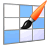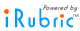# iRubric: Fractions on a number line rubric

 find rubric
 delete .rubinfoitem { padding:5px; white-space: nowrap; } Fractions on a number line  Rubric Code: F23A83C Ready to use Public Rubric Subject: Math   Type: Assessment   Grade Levels: K-5Number line fractions Fair 1 pts Good 2 pts Excellent 3 pts Conceptual understanding Student understands that fractions get bigger as you move from left to right across the number line Fair Fractions do not get bigger, or stay the same across the number line. Good Student will sometimes show fractions getting larger across the number line, or the denominator and not the numerator increases. Excellent The student consistently shows fractions getting larger across the number line. Conceptual understanding Student understands numerator and denominator Fair Student does not use correct numerator and denominator. Good Student can sometimes demonstrate understanding by using the correct numerator and denominator. Excellent Student writes fractions correctly showing that fractions represent parts of the whole. Conceptual Understanding Student shows understanding that fractions should be equal or even. Fair Student does not attempt to make tick marks even across the number line. Good Student sometimes makes tick marks even across the number line. Excellent Student consistently makes tick marks even across the number line. Problem solving Student correctly labels the fractions when tick marks are given Fair The student does not correctly label, or labels are missing. Good Student can sometimes correctly label when tick marks are given. Excellent Student can consistently label when tick marks are given. Problem Solving Student correctly makes tick marks Fair Student makes no tick marks. Good Students makes tick marks, but incorrectly or unevenly, and/or Student does not make tick marks on all number lines. Excellent Students correctly and evenly makes tick marks on all number lines. Problem Solving Student correctly labels fractions on the number line Fair Student does not correctly label fractions. No evidence of looking at the problem. Good Student partially labels fractions correctly. Fraction may have correct numbers, but numerator and denominator are transposed. Excellent Student correctly labels all fractions on the number line. Procedural Fluency Student can correctly label when the fractions change Fair Student writes the same fraction on the number line regardless of the given fraction Good Student can SOMETIMES correctly label the given fraction Excellent Student has correct fractions on correct number lines.

Subjects:

Types:

You may also be interested in:

Do more with this rubric:

Preview this rubric.

Modify this rubric.

Make a copy of this rubric and begin editing the copy.

Show a printable version of this rubric.

Add this rubric to multiple categories.

Bookmark this rubric for future reference.
Assess

Test this rubric or perform an ad-hoc assessment.

Build a gradebook to assess students.

Apply this rubric to any object and invite others to assess.
Share

Email this rubric to a friend.

Discuss this rubric with other members.

Do more with rubrics than ever imagined possible.

Only with iRubrictm.